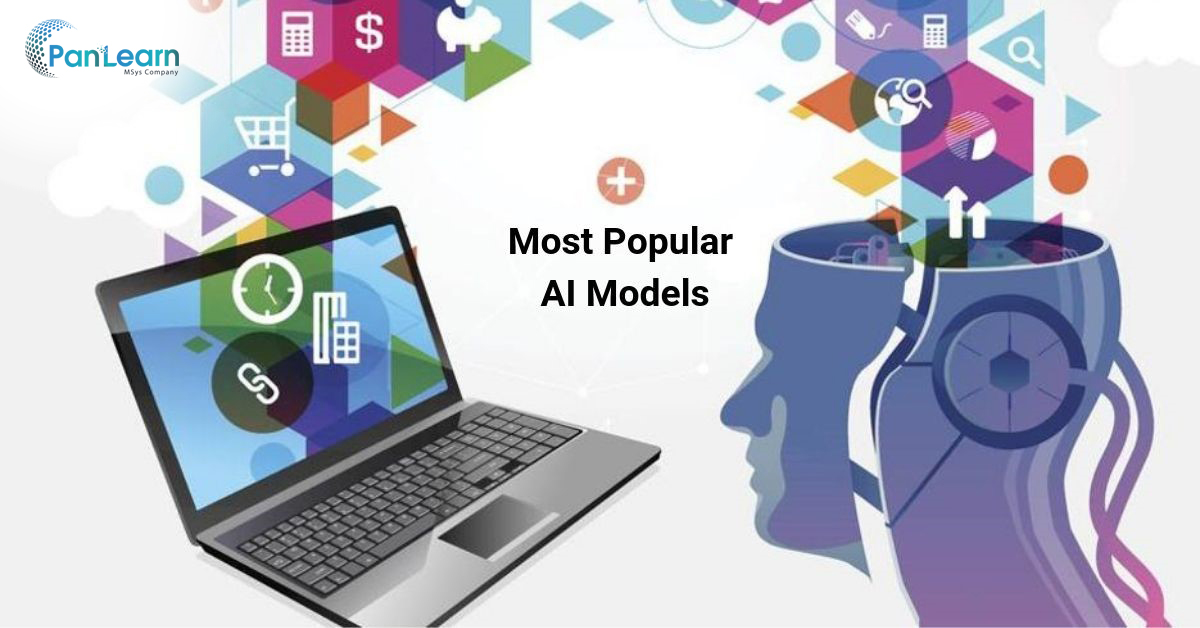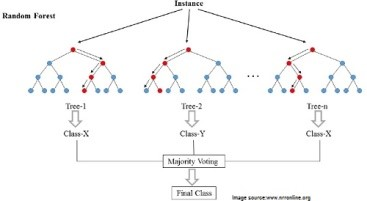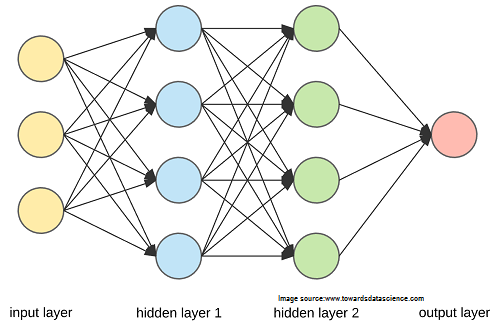# Most Popular AI Models

12816By John White Last Updated on Jun 14, 2021
•• Categories AI & Machine Learning

Learning Vector Quantization

The only major downside of KNN is the need to store and update huge datasets. Learning Vector Quantization or LVQ is the evolved KNN model, the neural network that uses the codebook vectors to define the training datasets and codify the required results. Thus said, the vectors are random at first, and the process of learning involves adjusting their values to maximize the prediction accuracy.

Thus said, finding the vectors with the results of the most similar value in the highest degree of accuracy of predicting the value of the outcome.

Support Vector Machines

This algorithm is one of the most widely discussed among data scientists, as it provides very powerful capabilities for data classification. The so-called hyperplane is a line that separates the data input nodes with different values, and the vectors from these points to the hyperplane can either support it (when all the data instances of the same class are on the same side of the hyperplane) or defy it (when the data point is outside the plane of its class).

Suggested Read: Top artificial intelligence technologies

The best hyperplane would be the one with the largest positive vectors and separating most of the data nodes. This is an extremely powerful classification machine that can be applied to a wide range of data normalization problems.

Random Decision Forests or BaggingRandom decision forests are formed of decision trees, where multiple samples of data are processed by decision trees and the results are aggregated (like collecting many samples in a bag) to find the more accurate output value.

Instead of finding one optimal route, multiple suboptimal routes are defined, thus making the overall result more precise. If decision trees solve the problem you are after, random forests are a tweak in the approach that provides an even better result.

Deep Neural NetworksDNNs are amongst the most broadly used ML and AI algorithms. There are significant improvements in deep learning-based text and speech apps, deep neural networks for machine perception and OCR, as well as using deep learning to empower reinforced learning and robotic movement, along with other miscellaneous applications of DNNs.

Final Thoughts Most Popular AI Algorithms

There is a variety of AI algorithms and ML models. Some are well appropriate for data classification, some excel in other areas. No model fits all sizes, so choosing the best one for your case is essential. How to know if this model is the right one? Consider the following factors:

• The 3 V’s of Big Data you have to process (volume, variety, and velocity of input)
• The number of computing resources at your disposal
• The time you can spend on data processing
• The goal of data processing

So, if a few models offer 94% prediction correctness at the cost of double longer processing time, as associated with an 86% precise algorithm — the variety of options grows greatly.

However, the biggest problem usually is the general lack of high-level expertise needed to design and implement the data analysis and Machine learning solution. This is why most of the businesses choose one of Managed Services Providers specializing in Big Data and AI solutions.